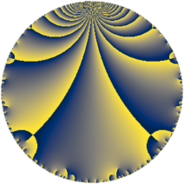# Properties

 Label 162.4.aLevel $162$ Weight $4$ Character orbit 162.a Rep. character $\chi_{162}(1,\cdot)$ Character field $\Q$ Dimension $12$ Newform subspaces $8$ Sturm bound $108$ Trace bound $5$

# Related objects

## Defining parameters

 Level: $$N$$ $$=$$ $$162 = 2 \cdot 3^{4}$$ Weight: $$k$$ $$=$$ $$4$$ Character orbit: $$[\chi]$$ $$=$$ 162.a (trivial) Character field: $$\Q$$ Newform subspaces: $$8$$ Sturm bound: $$108$$ Trace bound: $$5$$ Distinguishing $$T_p$$: $$5$$

## Dimensions

The following table gives the dimensions of various subspaces of $$M_{4}(\Gamma_0(162))$$.

Total New Old
Modular forms 93 12 81
Cusp forms 69 12 57
Eisenstein series 24 0 24

The following table gives the dimensions of the cuspidal new subspaces with specified eigenvalues for the Atkin-Lehner operators and the Fricke involution.

$$2$$$$3$$FrickeDim.
$$+$$$$+$$$$+$$$$3$$
$$+$$$$-$$$$-$$$$3$$
$$-$$$$+$$$$-$$$$2$$
$$-$$$$-$$$$+$$$$4$$
Plus space$$+$$$$7$$
Minus space$$-$$$$5$$

## Trace form

 $$12 q + 48 q^{4} + 24 q^{7} + O(q^{10})$$ $$12 q + 48 q^{4} + 24 q^{7} - 36 q^{10} - 30 q^{13} + 192 q^{16} - 30 q^{19} + 36 q^{22} + 822 q^{25} + 96 q^{28} + 132 q^{31} + 216 q^{34} - 678 q^{37} - 144 q^{40} - 1434 q^{43} + 504 q^{46} - 72 q^{49} - 120 q^{52} - 324 q^{55} + 1332 q^{58} - 1002 q^{61} + 768 q^{64} - 2838 q^{67} - 288 q^{70} + 2184 q^{73} - 120 q^{76} + 780 q^{79} - 1980 q^{82} + 3294 q^{85} + 144 q^{88} - 1140 q^{91} - 3096 q^{94} - 6078 q^{97} + O(q^{100})$$

## Decomposition of $$S_{4}^{\mathrm{new}}(\Gamma_0(162))$$ into newform subspaces

Label Dim $A$ Field CM Traces A-L signs $q$-expansion
$a_{2}$ $a_{3}$ $a_{5}$ $a_{7}$ 2 3
162.4.a.a $1$ $9.558$ $$\Q$$ None $$-2$$ $$0$$ $$9$$ $$-31$$ $+$ $-$ $$q-2q^{2}+4q^{4}+9q^{5}-31q^{7}-8q^{8}+\cdots$$
162.4.a.b $1$ $9.558$ $$\Q$$ None $$-2$$ $$0$$ $$21$$ $$8$$ $+$ $+$ $$q-2q^{2}+4q^{4}+21q^{5}+8q^{7}-8q^{8}+\cdots$$
162.4.a.c $1$ $9.558$ $$\Q$$ None $$2$$ $$0$$ $$-21$$ $$8$$ $-$ $+$ $$q+2q^{2}+4q^{4}-21q^{5}+8q^{7}+8q^{8}+\cdots$$
162.4.a.d $1$ $9.558$ $$\Q$$ None $$2$$ $$0$$ $$-9$$ $$-31$$ $-$ $+$ $$q+2q^{2}+4q^{4}-9q^{5}-31q^{7}+8q^{8}+\cdots$$
162.4.a.e $2$ $9.558$ $$\Q(\sqrt{3})$$ None $$-4$$ $$0$$ $$-12$$ $$16$$ $+$ $-$ $$q-2q^{2}+4q^{4}+(-6+\beta )q^{5}+(8-2\beta )q^{7}+\cdots$$
162.4.a.f $2$ $9.558$ $$\Q(\sqrt{105})$$ None $$-4$$ $$0$$ $$-9$$ $$19$$ $+$ $+$ $$q-2q^{2}+4q^{4}+(-5-\beta )q^{5}+(9-\beta )q^{7}+\cdots$$
162.4.a.g $2$ $9.558$ $$\Q(\sqrt{105})$$ None $$4$$ $$0$$ $$9$$ $$19$$ $-$ $-$ $$q+2q^{2}+4q^{4}+(4-\beta )q^{5}+(10+\beta )q^{7}+\cdots$$
162.4.a.h $2$ $9.558$ $$\Q(\sqrt{3})$$ None $$4$$ $$0$$ $$12$$ $$16$$ $-$ $-$ $$q+2q^{2}+4q^{4}+(6+\beta )q^{5}+(8+2\beta )q^{7}+\cdots$$

## Decomposition of $$S_{4}^{\mathrm{old}}(\Gamma_0(162))$$ into lower level spaces

$$S_{4}^{\mathrm{old}}(\Gamma_0(162)) \cong$$ $$S_{4}^{\mathrm{new}}(\Gamma_0(6))$$$$^{\oplus 4}$$$$\oplus$$$$S_{4}^{\mathrm{new}}(\Gamma_0(9))$$$$^{\oplus 6}$$$$\oplus$$$$S_{4}^{\mathrm{new}}(\Gamma_0(18))$$$$^{\oplus 3}$$$$\oplus$$$$S_{4}^{\mathrm{new}}(\Gamma_0(27))$$$$^{\oplus 4}$$$$\oplus$$$$S_{4}^{\mathrm{new}}(\Gamma_0(54))$$$$^{\oplus 2}$$$$\oplus$$$$S_{4}^{\mathrm{new}}(\Gamma_0(81))$$$$^{\oplus 2}$$